1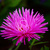```var maxTime = 30*60; // seconds
var time = maxTime;
\$('body').on('keydown mousemove mousedown', function(e){
time = maxTime; // reset
});
var intervalId = setInterval(function(){
time--;
if(time <= 0) {
clearInterval(intervalId);
}
}, 1000)
// 提示用户
// 该干嘛干嘛
}```0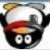0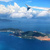0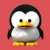0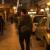```(function () {
var startTime = (new Date).getTime(),  //进入页面时间
interval = 0,  //进入页面到现在间隔了多少秒
clock;

//每500毫秒计算一次
clock = setInterval(function () {
interval = Math.ceil(((new Date).getTime() - startTime) / 1000);
if (interval > 60 * 30) {
clearInterval(clock);
callback();
}
}, 500);

function callback() {